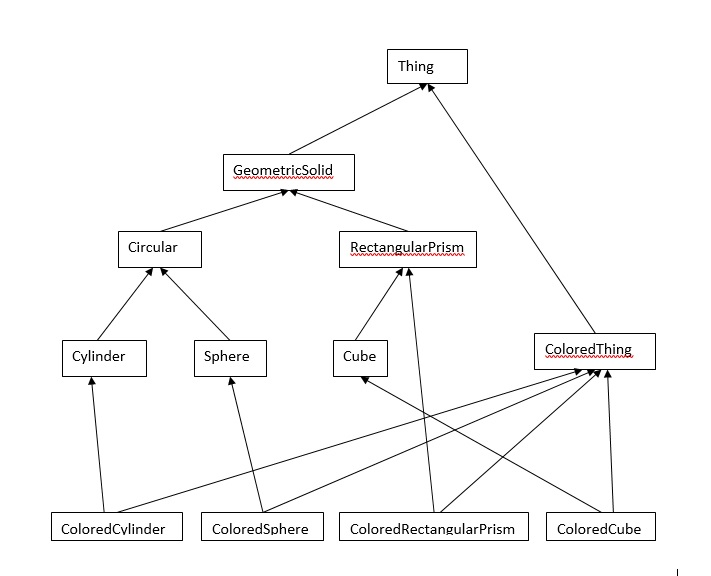## Assignment 4 - Binary Data and Multiple Inheritance

### due Tuesday, 2/9   1:30 pm

This assignment will give you practice processing a binary file, manipulating binary data, using casts, and multiple inheritance.  The assignment has two parts - processing a binary file and working with the 12-class multiple  inheritance hierarchy shown below.  You should only turn in one (set of) source code that contains both parts.

Input File: ass4data.bin

### Part 1 - Read and interpret the binary input file

The binary input file contains data that will be used to create geometric of the types listed below.  There are 50 records in the input file, consisting of 8 different types.  Each type represents data for one of the non-abstact class objects described below.  You should first complete part 1 of the assignment to make sure that you can correctly read and process the input file.  To read each read record, follow these steps:
1. Read one byte from the binary file.  That byte is coded and contains the type and color of each geometric solid.
2. Toggle the first and last bits of the one byte that you read.
3. Split the resulting byte into two nibbles.  The first nibble contains the type of geometric solid.  The second nibble contains the color (if any) of the geometric solid.  See the (nibble codes) codes below for the solid type and color.  Determine the type and color (if any) of the solid and read in the correct number of double values to define Thing.  Use the partial text output to verify the processing of the binary file.
 Geometric Solid Type Code (first nibble value) Color Code (second nibble value) 1 = cylinder 2 = sphere 4 = rectangular prism 8 = cube 0 = No color 1 = red 2 = blue 4 = green 8 = yellow

#### Interpretation of the binary file

Use this partial output to make sure you are corrected reading and interpreting the binary file.  You do not have to submit this output.  It is to be used for your verification of part 1.  This data is the input for part 2, described below.

 1st Byte Toggled   -Nibbles-   -------- Doubles ----------      147    0x12   0x1   0x2   3.41176   1.13333   4.36842      161    0x20   0x2     0   1.46154      201    0x48   0x4   0x8   5.60000   5.00000   9.60000   3.45455        1    0x80   0x8     0   7.54545        0    0x81   0x8   0x1   2.53846   1.80000      160    0x21   0x2   0x1   2.64706   3.83333        0    0x81   0x8   0x1   9.60000   8.16667      201    0x48   0x4   0x8   3.10000   1.76471   2.42857   2.50000      193    0x40   0x4     0   1.68421   3.40000   4.88889 ...

### Part 2 - Multiple Inheritance### Class Descriptions

1. Thing – Abstract, contains a function, name() that returns the class name.
2. GeometricSolid – Abstract, contains a pure virtual function, volume().
3. Circular – Abstract, contains a floating-point radius data member.
4. RectangularPrism – contains 3 floating-point data members, define the volume function in this class.
5. ColoredThing – Abstract, contains string and double data members to store the color and weight.  Contains a pure virtual function, density().
6. Cylinder – Derived from Circular.  Contains a double, height member.  Define the volume function for this class.
7. Sphere – Derived from Circular.  Define the volume function for this class.
8. Cube – Derived from RectangularPrism.
9. ColoredCylinder – Derived from ColoredThing and Cylinder.  Define density as weight divided by volume for this class.
10. ColoredSphere – Derived from ColoredThing and Sphere.  Define density as weight divided by volume for this class.
11. ColoredRectangularPrism – Derived from ColoredThing and RectangularPrism.  Define density as weight divided by volume for this class.
12. ColoredCube – Derived from ColoredThing and Cube.  Define density as weight divided by volume for this class.
Every class must be able to identify itself (with a name function).  There are 8 non-abstract classes.

Class Data

 Thing                    Binary data   Representing Cylinder                 2 doubles     radius and height Sphere                   1 double      radius RectangularPrism         3 double      length, width, and height Cube                     1 double      side ColoredCylinder          3 doubles     radius, height, weight, color ColoredSphere            2 doubles     radius, weight, color ColoredRectangularPrism  4 doubles     length, width, height, weight, color ColoredCube              2 doubles     side, weight, color

### Requirements

1. Read in the binary data file, correctly processing each particular type of Thing.
2. Using a pointer to a Thing, and making use of polymorphism, allocate memory dynamically for a specific type of GeometricSolid.  Don’t forget your delete.
3. Overload the insertion operator ( e.g. ostream& operator<<(ostream&, const GeometricSolid&)  ) for printing both GeometricSolid objects and ColoredThing objects.  Note: the ColoredCylinder, ColoredSphere, etc. will have to use both overloaded insertion operators.
4. Use the correct C++ cast types.  In particular, you should use a reinterpret_cast when reading in the binary file using the istream::read() function, and use a dynamic_cast to downcast a Thing pointer to utilize the correct operator<<() function call for printing either a GeometricSolid or a ColoredThing object.
5. The output should be produced by your overloaded insertion operators and match that which is partially displayed below.
6. Use the following formulas:
 Solid Volume Rectangular Solid V = l w h Sphere V = 4/3(pi)r3 Cylinder V = (pi)r2h Cube V = s3